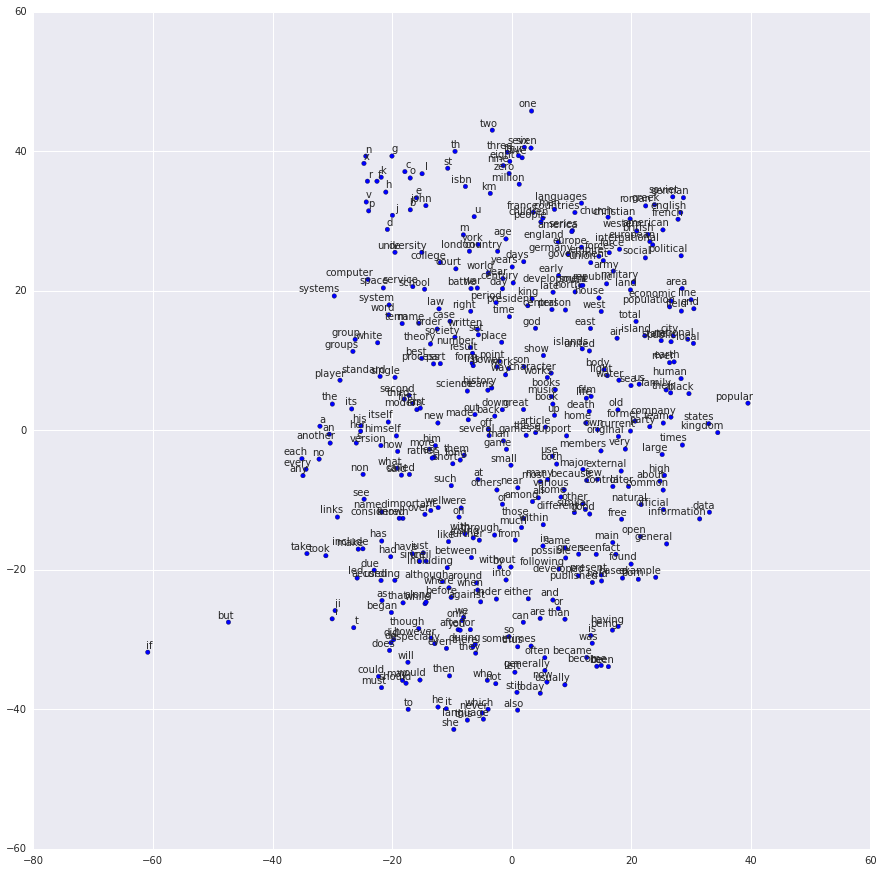# Assignment 1.3: Naive word2vec (40 points)¶

This task can be formulated very simply. Follow this paper and implement word2vec like a two-layer neural network with matrices $W$ and $W'$. One matrix projects words to low-dimensional 'hidden' space and the other - back to high-dimensional vocabulary space.## Results of this task: (30 points)¶

• trained word vectors (mention somewhere, how long it took to train)
• plotted loss (so we can see that it has converged)
• function to map token to corresponding word vector
• beautiful visualizations (PCE, T-SNE), you can use TensorBoard and play with your vectors in 3D (don't forget to add screenshots to the task)

## Extra questions: (10 points)¶

• Intrinsic evaluation: you can find datasets here
• Extrinsic evaluation: you can use these

Also, you can find any other datasets for quantitative evaluation.

Again. It is highly recommended to read this paper

Example of visualization in tensorboard: https://projector.tensorflow.org

Example of 2D visualisation: